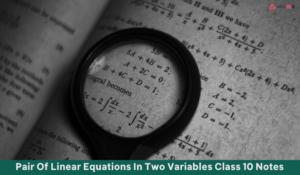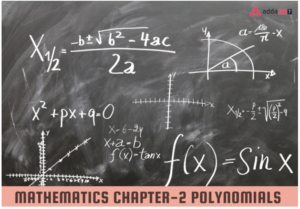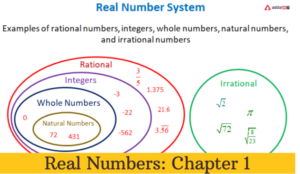Online Tution   »   Class 10   »   Arithmetic progression Class 10

# Arithmetic progression, Sum, Formula, Notes, Class 10 Ch-5

## What is Arithmetic Progression?

An arithmetic progression is a sequence of numbers, obtained by adding a fixed number to the preceding term starting from the first term such that the difference between each consecutive term remains the same.

Each of the numbers in the list is called a term and the fixed number is called the common difference of the AP which can be any integer.

For example: 2,5,8,11…. having a common difference of 3.

## Arithmetic Progression- General term of an AP

1. The general form of an AP is:

a ,a+d ,a+2d ,a+3d ,….,a+(n−1)d

1. An AP with a finite number of terms is called a finite AP having           a+(n−1)d as the last term.

For example:

Finite AP: 1,3,5,7,….,25

An AP which neither has a finite number of terms nor has a last term is called an infinite AP.

For example:

Infinite AP: 2,4,6,8…..∞

3 The nth term of the AP: an=a+(n−1)d, where a is the first term of the sequence and d is the common difference.

The Second term: a2=a+(2−1)d=a+d

Similarly, the third term a3=a+(3−1)d=a+2d

The fourth term a4=a+(4−1)d=a+3d and so on till the last term.

## Arithmetic Progression- Examples

Example 1:

An AP has a first term 3, common difference 4. Find the third and fifth term of the AP.

Ans:

a=3, d=4

a3=3+(3−1)4

a3=11

Similarly,

a5=3+(5−1)4

a5=19

4 nth term of an AP from the end:  tn=L−(n−1)d, where L is the last term of the AP.

Example 2:

An AP has a common difference 2 and last term 24. Find the fourth term of the AP from the end.

Ans:

d=2, L=24

t4=24−(4−1)2

t4=18

Sum of the terms of an AP:

Sum of n terms of an AP if first term and common difference is given:

S=n2(2a+(n−1)d)

Sum of n terms of an AP if first term and last term l is given:

S=n2(a+l)

Example 3:

Find the sum of the first 10 terms of the AP 1,4,7,10…..34.

Ans:

S=102(2×1+(10−1)3)

=5(2+27)

=5×29

=145

Class 10 students can now join the board exam preparation batch by Adda247 and ace their board exam.

## Arithmetic Progression Class 10

An arithmetic progression is a sequence of numbers that differ from each other by a common difference. For example, the sequence 3, 6, 9, 12, ….. is an A.P. with a common difference of 3.

Common Difference:

The difference between the two consecutive terms of an A.P. is known as the common difference. For example, in the sequence 3, 6, 9, 12…., the common difference is 3.

The classification of the common difference:

• Positive, when the A.P. is increasing.
• Zero, when the A.P. is constant.
• Negative, when the A.P. is decreasing.

## General Form of an Arithmetic Progression

Say the terms a1, a2, a3……an are in A.P. If the first term is ‘a’ and its common difference is ‘d’. Then, the terms can also be expressed as follows.

1st term a1 = a

2nd term a2 = a + d

3rd term a3 = a + 2d

Therefore, we can also represent arithmetic progressions as:

a, a + d, a + 2d, ……

This representation is called the general form of an Arithmetic Progression.

Finite and Infinite A.P.

In the finite A.P., the numbers of terms are finite, and the last term of the A.P. exists.

In the infinite A.P., the number of terms is infinite, and the last term of an A.P. doesn’t exist.

## Sum of Arithmetic Progressions

The sum of n terms of an A.P. with ‘a’ as its first term and ‘d’ as its common difference is given by:

Sn=n2(2a+(n−1)d)

Arithmetic Mean

Arithmetic Mean is simply the average of two numbers. If we have two numbers n and m, we can add a number L in between them so that the three numbers form an arithmetic sequence like n, L, m. In this case, the number L is the arithmetic mean of the numbers n and m. On the basis of the properties of Arithmetic Progression, we may say:

L – n = m – L, that is, the arithmetic mean of n and m.

L=n+m2

## Arithmetic Progression Formulas

Here we have provided all the arithmetic formulas in the table below for class 10 Students.

## Properties of Arithmetic Progressions Class 10 Notes

• If the same number is added or subtracted from each A.P. term, the resulting terms in the sequence are also in A.P. with the same common difference.If each term in A.P. is divided or multiplied by the same non-zero number, the resulting series is also in A.P.

• Three numbers x, y, and z will be an A.P. if 2y = x + z.\

• A series is an A.P. if the nth term is a linear expression.

• If we pick terms from the A.P. in the regular interval, these selected terms will also make an A.P.

• If the terms of an arithmetic progression are increased or decreased with the same amount, the resulting sequence will also be an arithmetic progression.

## Important Points on Arithmetic Progressions for Class 10

• If each term of the A.P. is increased, decreased, multiplied, or divided by the same non-zero constant, the resulting sequence would also be in A.P.

• In the A.P., the number of terms equidistant from start to end will be constant.

• In order to solve most of the problems related to A.P., the terms can be conveniently taken as:

• 3 Terms: (a – d), a, (a + d).

• 4 Terms: (a – 3d), (a – d), (a + d), (a + 3d).

Let’s connect via chat or call our senior expert counsellor at +91-9625869989 to learn more about the different streams and options available.

We would love it if we could add some of your insights. If you have a definite goal of scoring the highest marks, then you can resolve your doubts via our app/quizzes and youtube class  assistance (https://youtube.com/c/Adda247Class9and10)

Related Post:

Polynomials Class 10 Chapter 2

Pair of Linear Equations in two variables Class 10 Chapter 3

## Arithmetic progression- FAQs

Question 1 Is arithmetic progression easy?

Ans. The Arithmetic Progression is the most commonly used sequence in maths with easy to understand formulas.

Question 2 What is n in AP formula?

Ans. The sum of n terms of AP is the sum(addition) of first n terms of the arithmetic sequence. It is equal to n divided by 2 times the sum of twice the first term – ‘a’ and the product of the difference between second and first term-‘d’ also known as common difference, and (n-1), where n is the number of terms to be added.

Question 3 Which term of the AP is 81?

Ans. Therefore, the 35th term is -81.

Question 4 What is the formula of GP?

Ans. The formula to calculate the sum of the first n terms of a GP is given by: Sn = a[(rn – 1)/(r – 1)] if r ≠ 1and r > 1. Sn = a[(1 – rn)/(1 – r)] if r ≠ 1 and r < 1. The nth term from the end of the GP with the last term l and common ratio r = l/ [r(n – 1)].

Question 5 If the number of terms in a GP is not finite, then the GP is called infinite GP. The formula to find the sum to infinity of the given

Ans.  GP is: S ∞ = ∑ n = 1 ∞ a r n − 1 = a 1 − r ; − 1 < r < 1.

Question 6 What is the TN formula?

Ans. The formula for the nth term is given by: Tn = a + (n − 1)d = dn + (a − d) (2) where a and d are fixed and n is the variable (integer ≥ 1). This corresponds to y = mx + b where m and b are fixed and x variable.

Question 7 What is common ratio?

Ans. The common ratio is the number you multiply or divide by at each stage of the sequence. You can find it by dividing two consecutive pairs of terms.

Question 8 What is a constant ratio?

Ans. When you have points with a constant ratio it means that your y:x or x:y ratio is the same for all points. To find the y:x ratio for each point, you write your y value on the left side of the colon and your x value on the right side of the colon

Sharing is caring!

Thank You, Your details have been submitted we will get back to you.
•Pair Of Linear Equations In Two Variable...
•Polynomials- Degree, Definition, Zero Po...
•Real Numbers Class 10 Notes, MCQs, Extra...
•Manufacturing Industries CBSE Class 10 N...
•CBSE Notes Class 10 Geography Chapter 4 ...
•The Age of Industrialisation- Class 10 H...